American Journal of Science, Engineering and Technology
Volume 2, Issue 1, March 2017, Pages: 1-5

A Bi-objective VRPTW Model for Non-adjacent Products

Mohammad Hossein Sarbaghi Yazdi, Farhad Esmaeili

Department of Industrial Engineering, Mazandaran University of Science and Technology, Babol, Iran

Email address: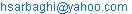(M. H. Sarbaghi Y.)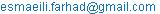(F. Esmaeili)

To cite this article:

Mohammad Hossein Sarbaghi Yazdi, Farhad Esmaeili. A Bi-objective VRPTW Model for Non-adjacent Products. American Journal of Science, Engineering and Technology. Vol. 2, No. 1, 2017, pp. 1-5. doi: 10.11648/j.ajset.20170201.11

Received: October 30, 2016; Accepted: December 26, 2016; Published: January 12, 2017

Abstract: Vehicle Routing Problem (VRP) with time windows is a generalization of the classic VRP. Specifically, every customer must be met in a certain time window. Sometimes in the real life, it is not possible to carry different products simultaneously. In other words, these products are non-adjacent. This paper presents a comprehensive model for the vehicle routing problem with time windows and the possibility of delivery split of non-adjacent products. The proposed model is an extension of VRP considering the profit in a bi-objective optimization model.

Keywords: VRP, Time Window, Bi-objective, Non-adjacent Products

Contents

1. Introduction

Today, transportation planning is one of the important fields in operations research, industrial engineering, and civil engineering. The main objective of this field is to minimize the cost of transportation of goods and materials from the producers to the consumers.

1.1. Vehicle Routing Problem

VRP is one of the important problems has been raised in the field of logistics management and transportation systems. VRP is a generalization of traveling salesman problem (TSP), which is considered as a vehicle. VRP is aimed at determining the optimum route of the vehicle so that to meet the demands of all customers where all constraints are satisfied and transportation cost is minimized. In classic VRP, the start and the end of all routes is a specific warehouse or a central depot.

The VRP is generally defined on a complete graph G=(V, A, D). Where V={V0, V1, V2, …, Vn} is the set of nodes (vertices) and A={(Vi, Vj): ij} is set of arcs that connect node i to j. V0 indicates central depot and Vn represents the customer n. dij represents distance or cost between node i and node j. qi is demand in node i. Typically, the objective is to minimize the total travel cost.

Dantzig and Ramser  proposed the VRP for the first time. After that, many variants of VRP have been considered: VRP with time windows (VRPTW), pickup and delivery (VRPPD), multi depot VRP (MDVRP), and so on.

1.2. Vehicle Routing Problem with Time Window

If each customer must be visited during a specific time window, we face with VRPTW. In fact, time window is a time interval with earliest time (bi) and the latest time (ei) that a customer permits to start receiving service. Some of applications of the VRPTW include postal deliveries, refuse collection, bank deliveries, school bus routing, etc.

Over the past 25 years, many researchers have worked on VRP with time windows. VRPTW belongs to NP-Hard problem , the same as the classic VRP, so many meta-heuristics have been used to solve it, e.g. Simulated Annealing (SA) [3, 4], Genetic Algorithms (GA) [5, 6], Tabu Search (TS) [7, 8] and Ant colony Optimization (ACO) . Some authors like Yu et al.  presented a hybrid approach to solve VRPTW, a mixture of ACO and TS. Küçükoglu and Öztürk  presented a hybrid algorithm which includes tabu search and simulated annealing.

In the last three decades, researchers have mixed VRPTW with other modes that are close to real-word problems, for instance VRPTW with Split Delivery (VRPTWSD) [12, 13]. Dror and Trudeau  presented split delivery problem for the first time. In VRPSD, unlike the classic VRP, more than one vehicle can supply demand of each node . El-Sherbeny  has reviewed literatures in VRPTW and its combination to other VRP modes. He studied various methods of solving VRPTW.

1.3. Vehicle Routing Problem with Profit

In classic VRP and TSP each node should meet once and it will fulfill the demand of all nodes, but in TSP with profit (TSPP) and VRP with profit (VRPP), there is not any obligation to serve all nodes and customers are served in accordance with the profit of meeting. In this problem, each node gets a reward after meeting and a trade-off between cost and profit in each node will indicate which nodes must been met . In TSPP and VRPP there are two objectives having obviously conflict:

Maximizing profit through meeting the maximum number of customers, which cause increase in distances and travel costs.

Minimizing travel distances that cause decrease in profit of customer’s meeting.

If two objectives were homogenous, for example both be currency, we can solve them via converting to the single-objective problem. As keller and Goodchild  mentioned, in many cases these two objectives are not commensurable and we should consider them as a bi-objective problem.

Feillet et al.  describe three classes of single-objective problems that have proposed in TSPP:

PTP (Profitable Tour Problem): their objective function is a combination of two objectives, i.e. minimizing travel cost minus profit of customers’ meeting .

OP (Orienteering Problem) or STSP (Selective TSP): their objective function is maximizing collected profit such that travel costs do not exceed a predetermined value Cmax .

QTSP (Quota TSP) or PCTSP (Prize Collecting TSP): their objective function is minimizing the total traveling distance such that profit of customers’ meeting which should not be less than a fixed amount of Pmin [21, 22].

It seems that VRPP has been less considered in the literature of VRP . Team OP (or TOP) is an extension of OP that has been studied by Chao et al. . They presented a five-steps metaheuristic approach based on simulated annealing to solve this problem. Lin and Yu  used a simulated annealing heuristic for TOP. Boussier et al.  used an exact method to solve this problem. Aráoz et al.  solved a Prize Collecting Rural Postman in which the objective is minimizing the residuum of collected profit and traveling costs.

2. Proposed Model

This paper presents a bi-objective model for VRP with time windows and split deliveries. The considered objectives are:

To maximize the profit of customers’ meeting: it considers specific profit for each unit of product which has been met.

To minimize the costs, e.g. traveling cost, waiting time cost, or vehicle cost.

In fact, presented model is a kind of developed VRPP.

As mentioned before, the considered two objectives are not homogenous. In real life, various routes are not the same in view of transportation complexity. For instance, distance between customers 2 and 8 is similar to distance between costumers 4 and 6, but traveling time of these two routes are not the same due to their path conditions, like speed constraint, traffic, etc. Therefore, for each arc between two nodes we consider a difficulty factor and a rest time depending on the traveling time. The time that each vehicle services a node depends on type and amount of its demand.

2.1. Assumptions and Characteristics of Model

All vehicles start from central depot and traverse a subset of customers in a specified sequence. Then they return to the central depot.

Each vehicle meets a customer for once.

Delivery split is possible so that demand of each customer (node) can be met by several vehicles.

Each customer has a time window to receive services.

If the vehicle arrives earlier than the lower bound of time window, it should wait until starting of service time.

A penalty is considered for vehicle tardiness in each node.

The number of available vehicles is given and vehicle costs are assumed to be zero if they are not in use.

The time that each vehicle serves a node (customer) depends on type and amount of demand which is met by the vehicle.

All vehicles have a constant speed and fixed cost per distance (kilometer or mile).

Difficulty factor of route is defined between 1 and 3.

The proposed model is designed for non-adjacent products and each customer has determined demand.

Each vehicle transports only predetermined product and it does not allow transporting any other product.

A defined award is considered for every unit of customer’s demand which has been met.

It is not obligatory to meet all the demands.

Each vehicle should rest when it receives to a node and the rest time depends on distance passed from the previous node to the current one.

2.2. Notations

The notation we used in our model is presented below:

Sets:

Q= {1, 2,…, i, …., N} demanding nodes (points) where point 1 denotes central depot.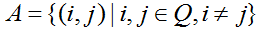arcs or connection between nodes

V= {1, 2, …, v, …., U} vehicles

K= {1, 2, …, r, …., O} products

Parameters:

Pir Profit of r-type product in node i

TC Traveling cost

dij Length of arc A where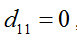,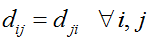and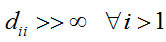bij Difficulty factor for route ij, so that bij=bji

tij Traveling time of arc A where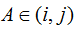pei Penalty for tardiness per each unit of time in node i

arv Binary variable which is equal to 1 if vehicle v is allowed to transport r-type product, and 0 otherwise.

qir Demand of node of i for product of type r.

gr Necessary time to serve each product of type r.

e Rest time that depends on passed distance

lbi Earliest possible time to start traveling from node i (lower bound of time window)

ubi Latest possible time to arrive in node i (upper bound of time window)

R Fixed cost of using each vehicle

Ve Average speed of each vehicle

M An enough huge number (M>>∞)

Decision variables:

xijv Binary variable which is equal to 1 if vehicle v goes from node i to j, 0 otherwise.

firv Shortage of demand of r-type product in node i that will be met by vehicle v.

wiv Waiting time of vehicle v in node i

hiv The time that vehicle v spends to serve in node i

reiv The time that vehicle v rests in node i

siv The time that vehicle v start to serve in node i

2.3. Mathematical Model

The proposed mathematical model is a bi-objective optimization model represented by equations (1)-(15).

The first objective function maximizes the profit of meeting of nodes (customers):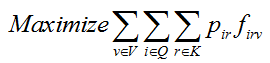(1)

The second objective function minimizes sum of three elements: 1) total passed distance, 2) fixed cost of using vehicles, and 3) total tardiness penalties in nodes: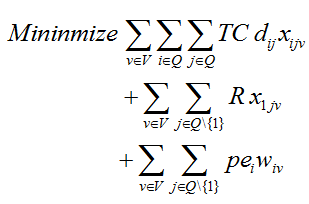(2)

Equation (3) expresses each vehicle cannot visit a node more than once.(3)

Equation (4) guaranties each vehicle exit after arriving in a node.(4)

Equation (5) assures if vehicle v supplies a portion of demand of type r in node i, it definitely will meet node i.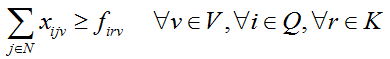(5)

The following equation shows vehicle v can supply demand of type r in node i.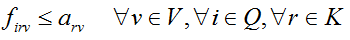(6)

Each vehicle cannot serve more than its capacity: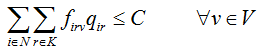(7)

The following equation calculates the time which vehicle v serves node i.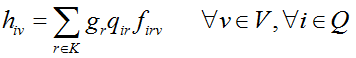(8)

Equation (9) calculates the rest time of vehicle v in node j(9)

The necessary time to traverse the arc of  is calculates by equation (10).(10)

Equations (11)-(13) are related to time window. Moreover, equations (11) and (12) prevent forming any subset tours.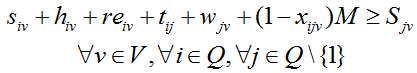(11)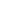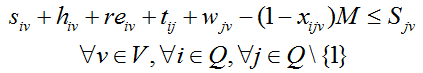(12)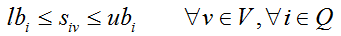(13)

Equations (14) and (15) declare the variable types and their signs.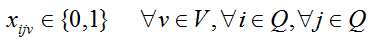(14)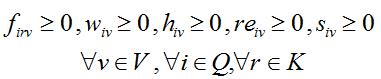(15)

3. Solving the Proposed Model with Augmecon Approach

A brief explanation of AUGMECON approach is presented here, an augmented version of the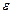-constraint method.

First, consider the following multi-objective problem: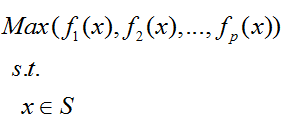(16)

Where S is the feasible region. In the-constraint method, one of the objective functions gets optimized while other objective functions are considered as constraints: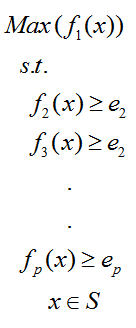(17)

We obtain different solution via changing the value of ei.

In AUGMECON, a developed version of-constraint method, lexicographic optimization is used to determine ei. After calculation of ei, difference between upper and lower bound is divided into several parts. The calculated values indicate allowable ei. Pareto-optimal solutions increase as the number of iterations increases. Then, the below function is solved using obtained values of ei.(18)

Where Si are slack variables and eps is a very small number (roughly between 10-3 and 10-6). For more information about AUGMECON approach we refer the interested readers to  and .

4. Computational Results

We used GAMS software to solve the presented model for 8 different randomly-generated instances. The results are shown in table 1.

Table 1. Computational results.

 Instance Instance characteristics Number of points on the Pareto-optimal frontier Computational time (seconds) Number of nodes Number of product types 1 4 2 10 111 2 4 3 10 244 3 5 2 10 412 4 5 3 10 921 5 6 2 55 1090 6 6 3 47 1909 7 7 2 45 7409 8 7 3 46 11208

Whereas the VRP belongs to NP-Hard problems, if the problem size (the number of parameters, variables and constrains) increases, the computational time will increase exponentially. So, it is not possible to solve large-dimension problems by GAMS software and heuristic or Meta-heuristic methods should be applied to solve these problems.

5. Conclusion

In this paper, a bi-objective optimization model is presented for VRPTW with split delivery to transport non-adjacent products. Moreover, several real-world issues are considered, i.e. difficulty of route, rest time (depending on passed distance) and serve time (depending on type and amount of demand). Finally, the presented model is solved using AUGMECON method in several instances and the results are discussed.

References

1. Dantzig, G. and Ramser, J.H. ‘The truck dispatching problem’, Management Science, Vol. 6, pp. 80-91.
2. Lenstra, J. and Rinnooy, K. ‘Complexity of vehicle routing and scheduling problems’, Networks, Vol.
3. Baños, R., Ortega, J., Gil, C., Fernández, A. and de Toro, F. (2013) ‘A Simulated Annealing-based parallel multi-objective approach to vehicle routing problems with time windows’, Expert Systems with Applications, Vol. 40, Issue 5, pp. 1696–1707.
4. Wang, C., Mu, D., Zhao, F. and Sutherland, J. W. (2015) ‘A parallel simulated annealing method for the vehicle routing problem with simultaneous pickup–delivery and time windows’. Computers & Industrial Engineering, Vol. 83, pp. 111–122.
5. Ursani, Z., Essam, D., Cornforth, D. and Stocker, R. (2011) ‘Localized genetic algorithm for vehicle routing problem with time windows’, Applied Soft Computing, Vol. 11, Issue 8, pp. 5375–5390.
6. Yang, B., Hu, Z.-H., Wei, C., Li, S.-Q., Zhao, L. and Jia, S. (2015) ‘Routing with time-windows for multiple environmental vehicle types’, Computers & Industrial Engineering, Vol. 89, pp. 150-161.
7. Belhaiza, S., Hansen, P. and Laporte, G. (2014) ‘A hybrid variable neighborhood tabu search heuristic for the vehicle routing problem with multiple time windows’. Computers & Operations Research, Vol. 52, pp. 269–281.
8. Li, X., Tian, P. and Leung, C. H. (2010) ‘Vehicle routing problems with time windows and stochastic travel and service times: Models and algorithm’, International Journal of Production Economics, Vol. 125, pp. 137–145.
9. Zare-Reisabadi, E. and Mirmohammadi, S.H. (2015) ‘Site dependent vehicle routing problem with soft time window: Modeling and solution approach’. Computers & Industrial Engineering, Vol. 90, pp. 177-185.
10. Yu, B., Yang, Z.Z. and Yao, B.Z. (2011) ‘A hybrid algorithm for vehicle routing problem with time windows’, Expert Systems with Applications, Vol. 38, pp. 435–441.
11. Küçükoglu, I., and Öztürk, N. (2015) ‘An advanced hybrid meta-heuristic algorithm for the vehicle routing problem with backhauls and time windows’, Computers & Industrial Engineering, Vol. 86, pp. 60-68.
12. Belfiore, P. and Yoshizaki, H.T.Y. (2013) ‘Heuristic methods for the fleet size and mix vehicle routing problem with time windows and split deliveries’, Computers & Industrial Engineering, Vol. 64, pp. 589–601.
13. Cherkesly, M., Desaulniers, G. and Laporte, G. (2015) ‘A population-based metaheuristic for the pickup and delivery problem with time windows and LIFO loading’, Computers & Operations Research, Vol. 62, pp. 23-35.
14. Dror, M. and Trudeau, P. (1989) ‘Savings by split delivery routing’, Transportation Science, Vol. 23, pp. 141–145.
15. Dror, M., Laporte, G. and Trudeau, P. (1994) ‘Vehicle routing with split deliveries’, Discrete Applied Mathematics, Vol. 50(3), pp. 239–354.
16. El-Sherbeny, N. (2010) ‘Vehicle routing with time windows: An overview of exact, heuristic and metaheuristic methods’, Journal of King Saud University (Science), Vol. 22, pp. 123–131.
17. Dell’Amico, M., Maffioli, F. and Varbrand, P. (1995) ‘On prize-collecting tours and the asymmetric travelling salesman problem’, International Transactions in Operational Research, Vol. 2, pp. 297–308.
18. Keller, C.P. and Goodchild, M. (1988) ‘the multi objective vending problem: A generalization of the traveling salesman problem’, Environment and Planning B: Planning and Design, Vol. 15, pp. 447-460.
19. Feillet, D., Dejax, P. and Gendreau, M. (2005) ‘Traveling salesman problems with profits’, Transportation Science, Vol. 36, pp. 188–205.
20. Laporte, G. and Martello, S. (1990) ‘The selective traveling salesman problem’, Discrete Applied Mathematics, Vol. 26, pp. 193–207.
21. Ausiello, G., Bonifaci, V. and Laura, L. (2008) ‘The online Prize-Collecting Traveling Salesman Problem’, Information Processing Letters, Vol. 107, pp. 199–204.
22. Pedro, O., Saldanha, R. and Camargo, R. (2013) ‘A Tabu Search Approach for the Prize Collecting Traveling Salesman Problem’, Electronic Notes in Discrete Mathematics, Vol. 41, pp. 261–268.
23. Archetti, C., Feillet, D., Hertz, A. and Speranza, M. G. (2010) ‘The undirected capacitated arc routing problem with profits’, Computers & Operations Research, Vol. 37, pp. 1860–1869.
24. Chao, I., Golden, B. and Wasil, E. (1996) ‘The team orienteering problem’, European Journal of Operational Research, Vol. 88, pp. 464–474.
25. Lin, S.-W. and Yu., V.F. (2015) ‘A simulated annealing heuristic for the multiconstraint team orienteering problem with multiple time windows’, Applied Soft Computing, Vol. 37, p.p. 632-642.
26. Boussier, S., Feillet, D. and Gendreau, M. (2007) ‘An exact algorithm for team orienteering problems’, 4OR, Vol. 5, pp. 211–230.
27. Aráoz, J., Fernández, E. and Meza, O. (2009) ‘Solving the prize-collecting rural postman problem’, European Journal of Operational Research, Vol.196, pp.886–896.
28. Mavrotas, G. (2009) ‘Effective implementation of the e-constraint method in Multi Objective Mathematical Programming problems’, Applied Mathematics and Computation, Vol.213, pp.455–465.
29. Mavrotas, G. and Florios, K. (2013) ‘An improved version of the augmented ε-constraint method (AUGMECON2) for finding the exact pareto set in multi-objective integer programming problems’, Applied Mathematics and Computation, Vol.219, pp. 9652–9669.

 Contents 1. 1.1. 1.2. 1.3. 2. 2.1. 2.2. 2.3. 3. 4. 5.
Article ToolsAbstractPDF(207K)
Follow on us
PUBLICATION SERVICE
JOIN US
RESOURCES
SPECIAL SERVICES
ADDRESS
Science Publishing Group
548 FASHION AVENUE
NEW YORK, NY 10018
U.S.A.
Tel: (001)347-688-8931